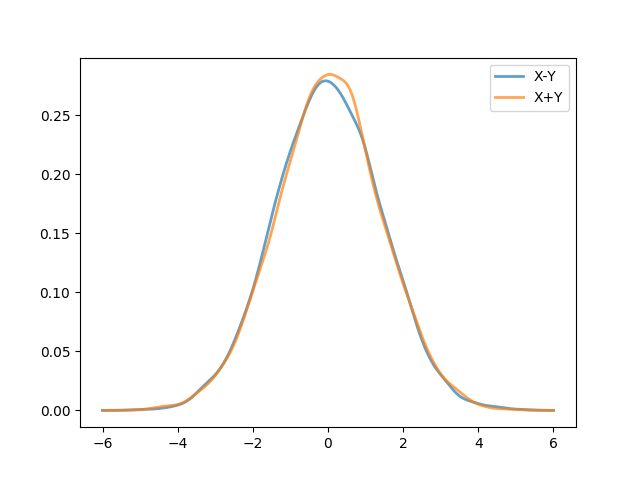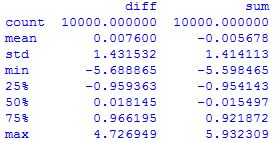# Simple But Evil #8 — Probability Distributions

Whether you’re teaching AP Stats or an introductory or senior level probability course for undergrads, you’ll know that students have a difficult time getting their minds wrapped around arithmetic for probability distributions. Here’s a simple example from the world of continuous probability.

Let $$X, Y$$ be iid normal with mean zero and variance 1. Now we’ll have a question like

What is the distribution of $$Z = X – Y$$?

Now a typical course will have students go through the math symbolism mechanics to eventually arrive at $$Z \sim N(0,\sqrt{2})$$, using the notation $$N(\mu,\sigma)$$ rather than $$\sigma^{2}$$. This is all good and well. But there’s often no visual follow up. Or even a sampling follow up.

Do me one favor, ask your students this simple but evil question.

Prove or disprove. Let $$X, Y$$ be iid normal with mean zero and variance 1. If $$Z_{1} = X – Y$$, $$Z_{2} = Y – X$$, and $$Z_{3} = X + Y$$ then $$Z_{1}$$, $$Z_{2}$$, and $$Z_{3}$$ all follow the same distribution.

I like this question for a lot of reasons. Here are a few main reasons (and there are lots of smaller reasons, too many to enumerate).

• Coursework in a probability course is often focused on transformations of distributions while the comparison of one distribution to another is held off for a statistics course. I see the wisdom in the separation, but that doesn’t mean we can’t start preparing students for what’s to come.
• This question can be a headscratcher because sometimes students want to believe that if “$$X$$ and $$Y$$ are the same thing” (that’s the mistake), then their difference should be zero. Ah, but $$X$$ and $$Y$$ are not the same thing. They just have the same distribution but come from two independent, though identically distributed “streams”. I say “streams” because what’s lost in a probability course is the sampling aspect of all these distributions — the thing left for a stats course. We are drawing from $$X$$ and drawing from $$Y$$. So the first draw from $$X$$ and from $$Y$$ don’t produce identical values. The $$X – Y$$ part of the question helps students to see the difference between that and $$X – X$$ or $$Y – Y$$.
• The second bullet point also helps separate the notion of “equality” when it comes to random numbers / processes. There’s equality in value and then there’s equality in distribution!
• Even if you don’t want to convert your probability course into a stats course, this is a great chance for visualizations!
• I will have a hard time believing that you won’t have a long discussion about this!
• Want a part deux? Compare $$\frac{X}{Y}$$ against $$\frac{Y}{X}$$.

### Some Visualizations

Here are some visualizations. [Also, an end of the post plug: remember to subscribe!]

Ok, first it’s good to just take a look at what $$X$$, $$Y$$, and $$X-Y$$ look like sampled (with a smoothed pdf overlaying them). (The histograms are normalized so they integrate to 1, rather than their height being their frequency.)Next, here are fits for $$X-Y$$ and $$X+Y$$ based on 10,000 samples.And finally, tables are visualizations too! ($$X-Y$$) is the “diff” column and ($$X + Y$$) is the “sum” column.